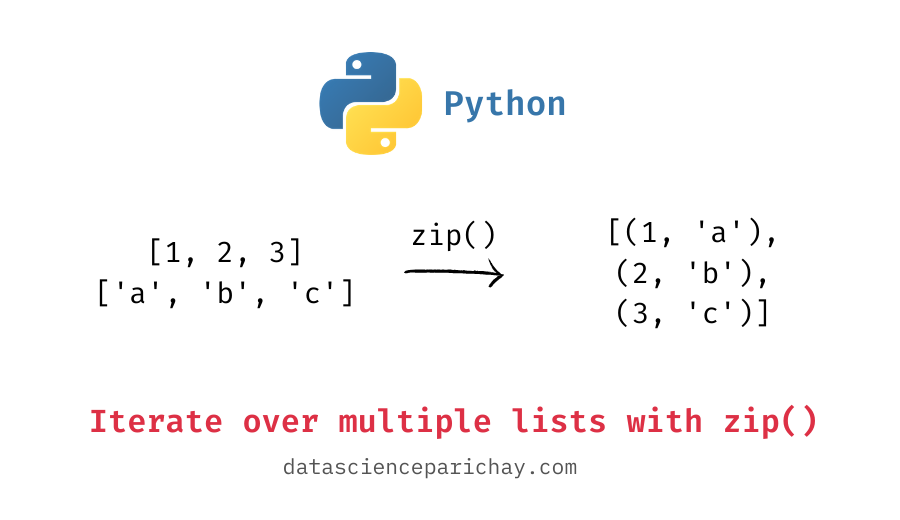# Python – Iterate over multiple lists in parallel using zip()

In this tutorial, we’ll look at how to iterate over multiple lists in parallel using the `zip()` function in python.

The `zip()` function in python is used to aggregate elements from multiple iterable objects element-wise into an iterable of tuples. For example, if you combine the lists `[1, 2, 3]` and `['a', 'b', 'c']` using the `zip()` function, it will return an iterable zip object which can be converted to a list of tuples – `[(1, 'a'), (2, 'b'), (3, 'c')]`. The following is the syntax to merge two lists:

```ls1 = [1, 2, 3]
ls2 = ['a', 'b', 'c']
combined_ls = list(zip(ls1, ls2))
print(combined_ls)```

Output:

`[(1, 'a'), (2, 'b'), (3, 'c')]`

To combine the lists, we pass each list as argument to the `zip()` function.

We can use the `zip()` function to iterate over multiple lists in parallel. For this, pass the lists as arguments to the function. The following example demonstrates this better.

Suppose we have two lists, one containing the names of students and the other containing their Math scores. You are given the task to print the names of students and marks next to each other.

One way of doing this is to iterate through the two lists in parallel using zip() and print the ith element in the first list and the ith element in the second list on each iteration.

```names = ['Sam', 'Meena', 'Jim', 'Emma', 'Steve']
scores = [57, 63, 61, 86, 72]
# iterate over both lists
for name, score in zip(names, scores):
print("{} : {}".format(name, score))```

Output:

📚 Data Science Programs By Skill Level

Introductory

Intermediate ⭐⭐⭐

🔎 Find Data Science Programs 👨‍💻 111,889 already enrolled

Disclaimer: Data Science Parichay is reader supported. When you purchase a course through a link on this site, we may earn a small commission at no additional cost to you. Earned commissions help support this website and its team of writers.

```Sam : 57
Meena : 63
Jim : 61
Emma : 86
Steve : 72```

You can see that names and scores of students are displayed together. The code above iterates over each element of both the lists and prints them together.

What would happen if you apply the `zip()` function on lists with different lengths?
Well, let’s find out.

```ls1 = [1, 2, 3]
ls2 = ['a', 'b']
combined_ls = list(zip(ls1, ls2))
print(combined_ls)```

Output:

`[(1, 'a'), (2, 'b')]`

When applied on lists with different lengths, the `zip()` stops at the end of the shortest list. Since the shortest list `ls2`, has length 2, the resulting list of tuples also has length 2.

Alternatively, you can use the `zip_longest()` function from the `itertools` module that stops at the end of the longest list and can fill the missing values with some custom value. The following example demonstrates its usage.

```from itertools import zip_longest
ls1 = [1, 2, 3]
ls2 = ['a', 'b']
# default
combined_ls = list(zip_longest(ls1, ls2))
print(combined_ls)
# pass the fill value
combined_ls = list(zip_longest(ls1, ls2, fillvalue='#'))
print(combined_ls)```

Output:

```[(1, 'a'), (2, 'b'), (3, None)]
[(1, 'a'), (2, 'b'), (3, '#')]```

First, we simply pass the two lists of lengths 2 and 3 to the `zip_longest()` function without specifying the fill value. Then, we passed the same two lists but specify the fill value to be `'#'`. Note that both the resulting lists are of length 3, that is, the length of the longest list.

For more on the python zip() function, refer to its documentation.

With this, we come to the end of this tutorial. The code examples and results presented in this tutorial have been implemented in a Jupyter Notebook with a python (version 3.8.3) kernel.

•